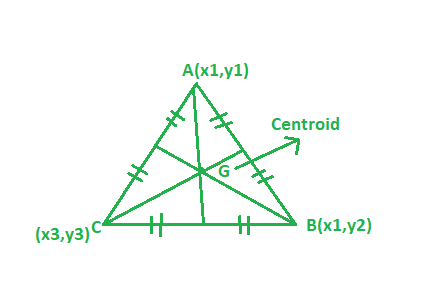# Program to find the Centroid of the triangle

Given the vertices of the triangle. The task is to find the centroid of the triangle:

Examples:

Input: A(1, 2), B(3, -4), C(6, -7)
Output: (3.33, -3)

Input: A(6, 2), B(5, -9), C(2, -7)
Output: (6.5, -9)


## Recommended: Please try your approach on {IDE} first, before moving on to the solution.Approach:Suppose if the vertices of a triangle are (x1, y1) (x2, y2) (x3, y3) then centroid of the triangle can be find from the following formula:## C++

 // CPP program to find the centroid of triangle  #include  using namespace std;     // Driver code  int main()  {      // coordinate of the vertices      float x1 = 1, x2 = 3, x3 = 6;      float y1 = 2, y2 = -4, y3 = -7;         // Formula to calculate centroid      float x = (x1 + x2 + x3) / 3;      float y = (y1 + y2 + y3) / 3;         cout << setprecision(3);      cout << "Centroid = "          << "(" << x << ", " << y << ")";         return 0;  }

## Java

 // Java program to find the centroid of triangle  import java.util.*;  import java.lang.*;     class GFG  {         // Driver code  public static void main(String args[])  {      // coordinate of the vertices      float x1 = 1, x2 = 3, x3 = 6;      float y1 = 2, y2 = -4, y3 = -7;         // Formula to calculate centroid      float x = (x1 + x2 + x3) / 3;      float y = (y1 + y2 + y3) / 3;         //System.out.print(setprecision(3));      System.out.println("Centroid = "         + "(" + x + ", " + y + ")");  }  }     // This code is contributed   // by Akanksha Rai(Abby_akku)

## Python 3

 # Python3 program to find   # the centroid of triangle      # Driver code       if __name__ == "__main__" :         # coordinate of the vertices       x1, x2, x3 = 1, 3, 6     y1, y2, y3 = 2, -4, -7            # Formula to calculate centroid       x = round((x1 + x2 + x3) / 3, 2)      y = round((y1 + y2 + y3) / 3, 2)         print("Centroid =","(",x,",",y,")")     # This code is contributed by ANKITRAI1

## C#

 // C# program to find the   // centroid of triangle  using System;     class GFG  {         // Driver code  static public void Main ()  {         // coordinate of the vertices      float x1 = 1, x2 = 3, x3 = 6;      float y1 = 2, y2 = -4, y3 = -7;             // Formula to calculate centroid      float x = (x1 + x2 + x3) / 3;      float y = (y1 + y2 + y3) / 3;             //System.out.print(setprecision(3));      Console.Write("Centroid = " +                     "(" + x + ", " + y + ")");  }  }     // This code is contributed   // by RaJ

## PHP

 

Output:

Centroid = (3.33, -3)


Don’t stop now and take your learning to the next level. Learn all the important concepts of Data Structures and Algorithms with the help of the most trusted course: DSA Self Paced. Become industry ready at a student-friendly price.

My Personal Notes arrow_drop_upCheck out this Author's contributed articles.

If you like GeeksforGeeks and would like to contribute, you can also write an article using contribute.geeksforgeeks.org or mail your article to contribute@geeksforgeeks.org. See your article appearing on the GeeksforGeeks main page and help other Geeks.

Please Improve this article if you find anything incorrect by clicking on the "Improve Article" button below.